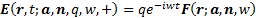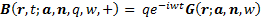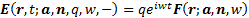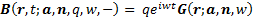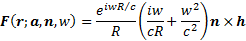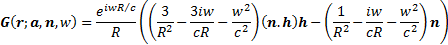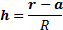/

### High resolution extended image near field optics 3. Exact radiating solutions to Maxwell’s equations in a vacuum

Next page

The explanation of the unusual properties of the idealised optical layout described in Section 2 lies in the behaviour of certain types of exact solutions of Maxwell’s equations in the presence of idealised plane mirrors.

Before exploring these further, let us first the nature of radiating solutions to Maxwell’s equations in a vacuum. These can be written as superpositions of (potentially infinitely many) outwardly and inwardly radiating electric and magnetic dipoles.

Born & Wolf (1980) describe the behaviour of a single outwardly radiating electric dipole, characterised by source location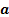, a unit vector,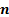, describing the direction in which the dipole is pointing, and an electric polarization vector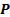whose value at point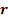and at timeis given by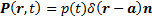, where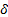is the Dirac function and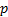is a function of time. The full (i.e. exact) solution to Maxwell’s equations (in a vacuum) for such a dipole then has the following form, where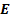,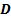,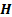and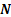are the electric field, electric displacement, magnetic and magnetic induction vectors respectively: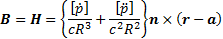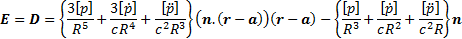Here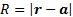andis the speed of light. Square brackets denote retarded values, i.e.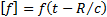.

The form of this solution is slightly easier to visualise in spherical polar coordinates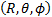taking the origin as the source location,,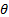as the angle betweenand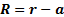and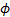as the angle that the projection of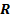onto the plane perpendicular tomakes with a constant vector perpendicular to. If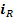,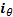and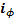are unit vectors in the direction of increasing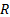,and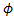respectively then the outwardly radiating electric dipole has the form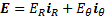and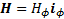where: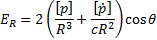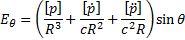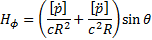The form of the inwardly radiating electric dipole, i.e. the time reversed solution, can be found by replacingby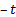and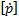by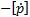(since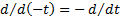) and by placing a negative sign in front of the corresponding expressions for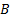and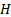(since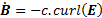).

The corresponding outwardly and inwardly radiating magnetic dipoles havereplaced by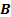andreplaced by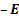, given the symmetric nature of Maxwell’s equations in a vacuum. For reasons that will become obvious later on, we will concentrate on these latter types of dipoles in the remainder of this analysis.

We can further decompose each of these dipoles into superpositions of sinusoidally time-varying dipoles all with the same origin, using Fourier analysis. These will be the types of dipoles that we will concentrate on in the remainder of this analysis. For magnetic dipoles with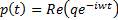,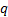and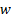constant, these have the following form (where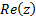is the real part of the complex numberandis the square root of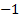):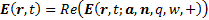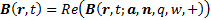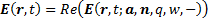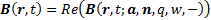where